# C++ program to Calculate Factorial of a Number Using Recursion

In this tutorial you will learn about the C++ program to Calculate Factorial of a Number Using Recursion and its application with practical example.

## C++ program to Calculate Factorial of a Number Using Recursion

In this tutorial, we will learn to create a C++ program that will Calculate Factorial of a Number Using Recursion using C++ programming.

## Prerequisites

Before starting with this tutorial we assume that you are best aware of the following C++ programming topics:

• Operators in C++ Programming.
• Basic Input and Output function in C++ Programming.
• Basic C++ programming.
• Conditional Statements in C++ programming.
• Arithmetic operations in C++ Programming.

## What is factorial?

The factorial means the product of the input number and its below integer value up to 1.

## Program to Calculate Factorial Using Recursion

Today, we will create a C++ program that will take the input number from the user and then will find the factorial for the input integer value using a recursion program.

## Output:-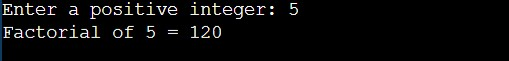In the above program, we have first initialized the required variable.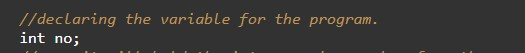• no = it will hold the integer value.
Taking input from the user the base number for finding factorial.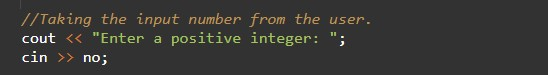Declaring the function.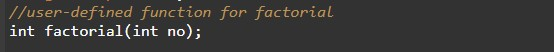Body of the function.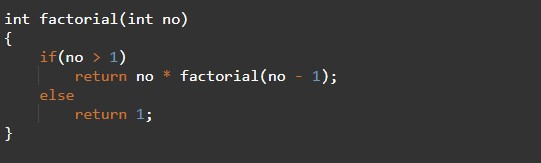Program Logic Code to print.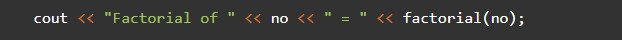In this tutorial we have learn about the C++ program to Calculate Factorial of a Number Using Recursion and its application with practical example. I hope you will like this tutorial.